Basics of Electromagnetism and special relativity

basic, electromagnetism, basics, field, magnetic, induction, electromagnetic, physics, science,

The theory of electromagnetism

Static magnetic field

Relativistic electromagnetism

Edward M. Purcell Electricity and Magnetism, Berkeley Physics Course, Vol. 2 in SI units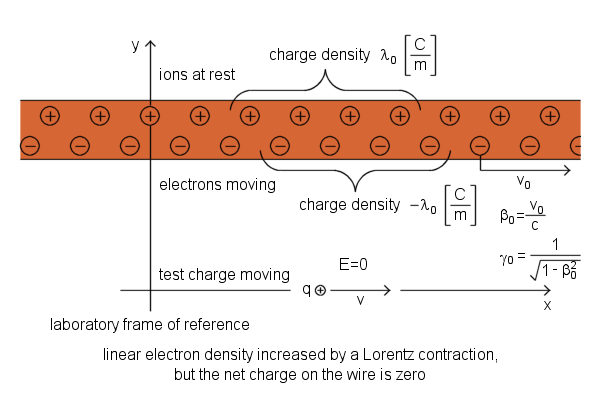Current-Carrying Wire, positive ions and electrons are shown separated for clarity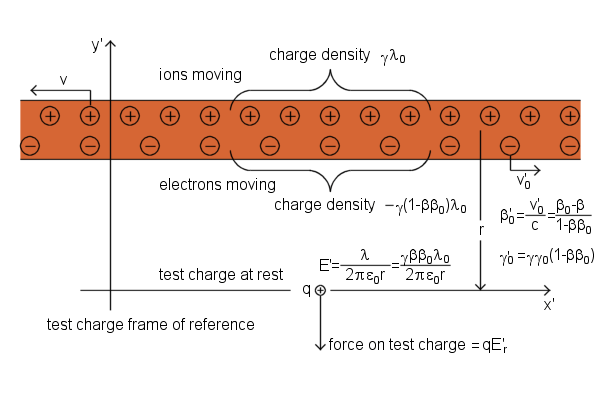Current-Carrying Wire, positive ions and electrons are shown separated for clarity

The linear density of positive charge in the test charge frame is
γλ0
Calculation of the linear density of negative charge in the test charge frame:
The electron linear density in the lab frame, which was - λ0, had already been increased by a Lorentz contraction.
Thus the linear density of negative charge in the electrons' own rest frame must have been
- λ00
We now calculate the speed of the electrons in the test charge frame in order to calculate their density there:
Relativistic formula for the addition of velocities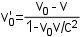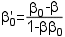Lorentz factorThe total linear density of charge in the wire in the test charge frameA radial electric field of infinite line chargeForce on test charge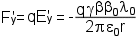For the observer in the test charge frame wire is length contractedThe total current I in the wire
- λ0v0 or - λ0β0c
Force on test charge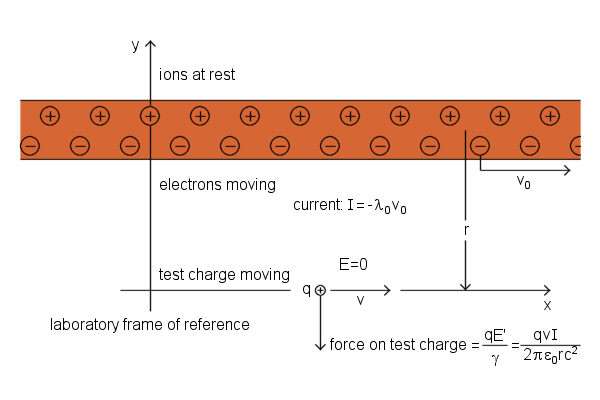Current-Carrying Wire, positive ions and electrons are shown separated for clarity

Interaction between a current-carrying wire and charge moving at right angle to the wire.

Edward M. Purcell Electricity and Magnetism, Berkeley Physics Course, Vol. 2 in SI units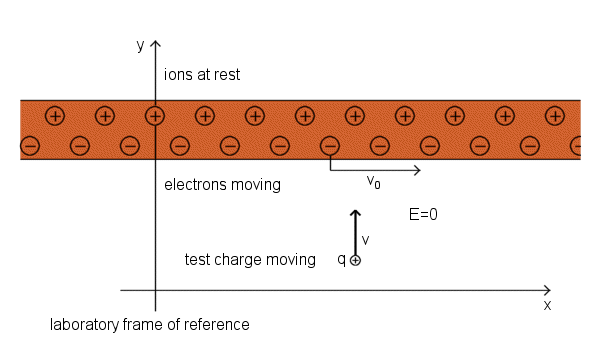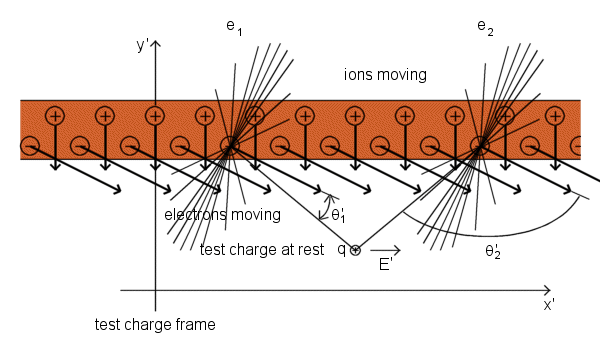Charge moving perpendicular to the wire experiences a force parallel to the wire, perpendicular to its direction of motion. Positive ions cannot cause a horizontal field at the test charge position. The x' component of the field from an ion on the left is exactly cancelled by the x' component of the field of a symmetrically positioned ion on the right. The effect we can see is caused by the electrons. Electrons are all moving diagonally in the test charge frame, downward and toward the right. Consider the two symmetrically located electrons e1 and e2. Their electric fields, relativistically compressed in the direction of the electrons' motion, are represented by a field lines. You can see that, although e1 and e2 are equally far away from the test charge, the field of electron e2 is stronger than the field of electron e1 at that location. That is because the line from e2 to the test charge is more nearly perpendicular to the direction of motion of e2. In other words, the angle θ' that appears in the denominator of equation for the magnitude of the field of electron in the frame moving relative to the electron is here different for e1 and e2, so that sin2θ'2>sin2θ'1. That is true for any symmetrically located pair of electrons on the line, as you can verify with the aid of picture. The electron on the right always acts stronger. Therefore, summing over all the electrons must give a resultant field E' in the x direction. The y' component of the electrons' field is exactly cancelled by the field of the ions. That E'y is zero is guaranteed by Gauss's law, for the number of charges per unit length of wire is the same as it was in the lab frame. The wire is uncharged in both frames. The force on the test charge, qE'x, transformed back into the lab frame is a force proportional to v in the x direction, which is the direction of v×B if B is a vector in the z direction, pointing out of the page.Radiation by an accelerated charge

Alternating magnetic field, electromagnetic radiation, electromagnetic induction

An electric field moves with the velocity that the particle had while emitting that field.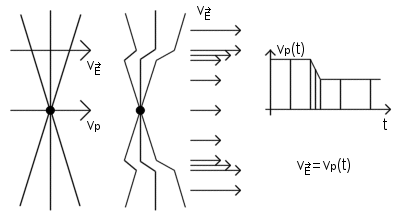Electric field of a charge that stops

Suppose a charge q has been moving at constant speed v0 in the x direction for a long time. Suddenly it stops after a short period of constant deceleration. Electric field of a charge travels radially outward and moves with the velocity that the charge had while emitting that field.

The velocity versus time graph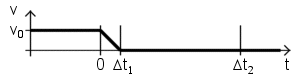The electric field

Assuming that the v0 ≪ c, we can neglect the relativistic compression of the field lines. Time t=0 it was the moment when deceleration began, and position x=0 it was the position of the particle at that moment. The particle has been moved a little farther on before coming to a stop, Δx=1/2*v0Δt1. That distance is very small compared with the other distances in the picture.

We now examine the electric field at a time t=Δt2≫Δt1. The electric field reaches a distance R=cΔt2. Thus, the field at a distance greater than R=cΔt2, in region I must be a field of a charge which has been moving and is still moving at the constant speed v0. That field appears to come from the point x=v0Δt2 on the x axis. That is where the particle would be now if it hadn't stopped. On the other hand, the field at a distance less than c(Δt2-Δt1), in region II must be a field of a charge at rest close to the position x=0 (exactly at x=1/2*v0Δt1).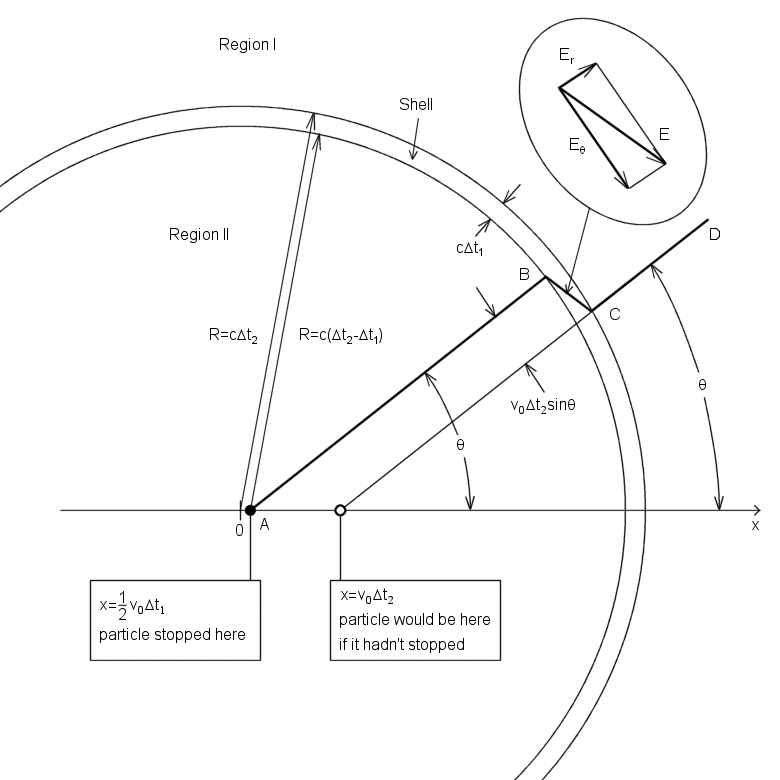What must the field be like in the transition region, the spherical shell of thickness cΔt1 between region I and region II? A field line segment AB lies on a cone around the x axis which includes a certain amount of flux from the charge q. Due to the Gauss' law, if CD makes the same angle θ with the axis, the cone on which it lies includes that same amount of flux. (Because the v0 ≪ c, we can neglect the relativistic compression of the field lines.) That's why AB and CD must be parts of the same field line, connected by a segment BC. The line segment BC shows us the direction of the filed E within the shell. This field E within the shell has both a radial component Er and a transverse component Eθ. From the geometry of the figure their ratio is easily found.(1)

Due to the Gauss' law, Er must have the same value within the shell thickness that it does in region II near B. That's why Er=q/4πε0R2=q/4πε0c2Δt22, and substituting this in Eq. 1 we obtain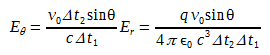(2)
And v0/Δt1 = a, the magnitude of the (negative) acceleration, and cΔt2= R, so our result can be written(3)

A remarkable fact is here revealed: Eθ is proportional to 1/R, not to 1/R2! As time goes on and R increases, the transverse field Eθ will eventually become very much stronger than Er. Accompanying this transverse (that is, perpendicular to R) electric field will be a magnetic field of equal strength perpendicular to both R and E. This is a general property of an electromagnetic wave.

We now calculate the energy stored in the transverse electric field above, in the whole spherical shell. The energy density is(4)
The volume of the shell is 4πR2cΔt1, and the average value of sin2θ over a sphere is 2/3. The total energy of the transverse electric field is consequently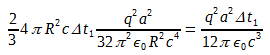(5)
To this we must add an equal amount for the energy stored in the transverse magnetic field:
Total energy in transverse electromagnetic field is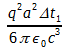(6)
The radius R has canceled out. This amount of energy simply travels outward, undiminished, with speed c from the site of the deceleration. Since Δt1 is the duration of the deceleration, and is also the duration of the electromagnetic pulse a distant observer measures, we can say that the power radiated during the acceleration process was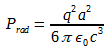(7)
As it is the square of the instantaneous acceleration that appears in Eq. 7, it doesn't matter whether a is positive or negative. Of course it ought not to, for stopping in one inertial frame could be starting in another. Speaking of different frames, Prad itself turns out to be Lorentz-invariant, which is sometimes very handy. That is because Prad is energy/time, and energy transforms like time, each being the fourth component of a four-vector. We have here a more general result than we might have expected. Equation 7 correctly gives the instantaneous rate of radiation of energy by a charged particle moving with variable acceleration-for instance, a particle vibrating in simple harmonic motion. It applies to a wide variety of radiating systems from radio antennas to atoms and nuclei.

Radiating Charge simulation - PhET INTERACTIVE SIMULATIONS

Purcell appendix B in the CGS system

Electromagnetic radiation of hydrogen atom

According to current theory, the electrons in the atoms occupy only the allowed energy levels and emit radiation only when they jump between energy levels, but it is only a simplification. Every accelerating charged particle emit electromagnetic radiation. Electrons continously emit and absorb electromagnetic radiation. By adding up the waves emitted by the hydrogen atom, we can see that the source is the expiring vibrations.electromagnetic radiation of hydrogen atom

The photoelectric effect

According to current theory, the beam of light is not a wave propagating through space, but rather a collection of discrete quantized wave packets (photons), each with energy hν, but it is only a simplification. The energy of light depends on the intensity of the electric field of the electromagnetic wave. The frequency of the electromagnetic wave depends on the velocity and acceleration of the electrons that emit it. The higher the frequency of the wave and the movement of electrons, the greater the accelerations affect the electrons, and thus the intensity of the electric field of the electromagnetic wave emitted by electrons is higher.photoelectric effect

Relativistic beaming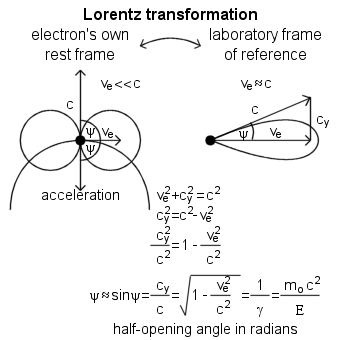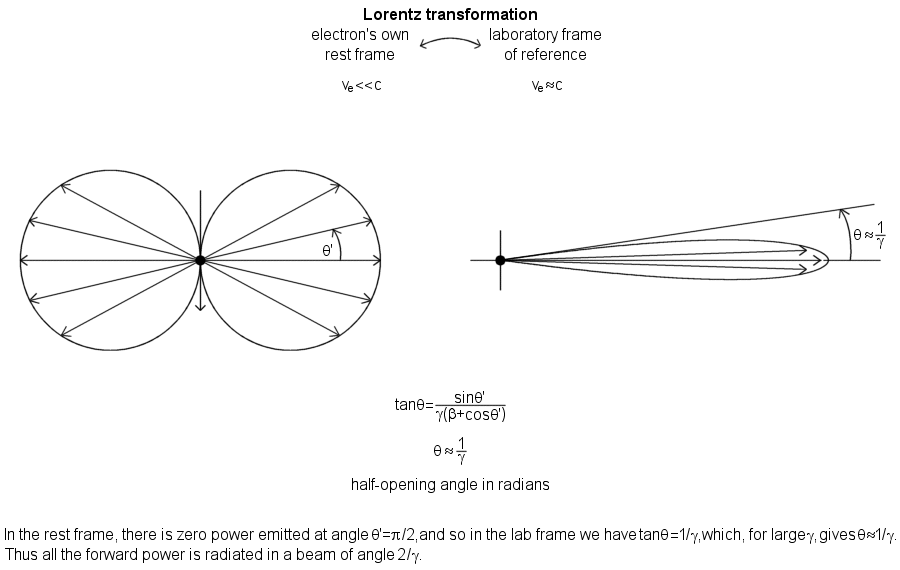Einstein relativistic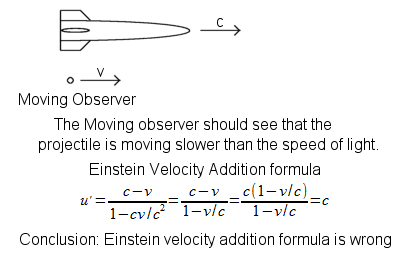Einstein relativistic

Matter Waves

de Broglie Matter Waves

Due to The Heisenberg's Uncertainty Principle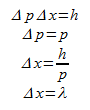Position uncertainty = Interparticle spacing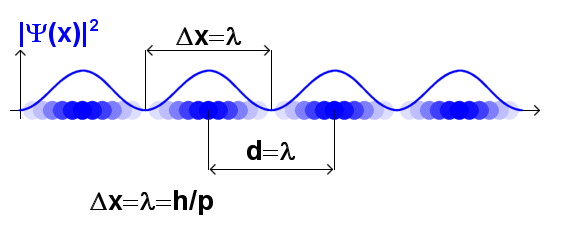de Broglie Matter Waves

Double-slit experiment

Feynman's double-slit experimentFeynman's double-slit experiment

Relativistic mass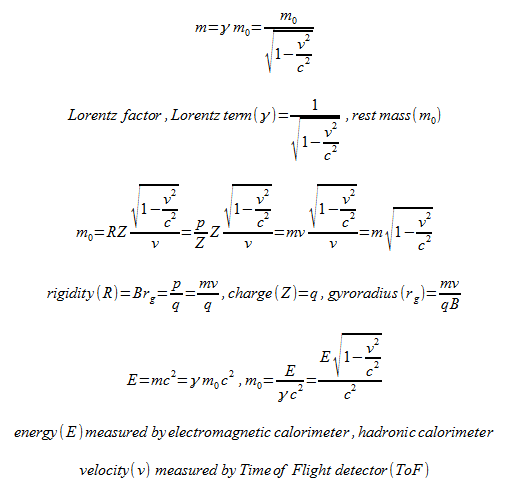relativistic mass

Relativistic momentumrelativistic momentum

Relativistic forcerelativistic force

Relativistic energyrelativistic energy

Mass defect

Beta particle mass

SymbolNameMass (u)Mass (MeV/c2)
e-electron0.0005490.51
e+positron0.0005490.51

Nuclear masses

SymbolNameMass (u)
nneutron1.008665
1
1
H, p
proton1.007276
2
1
D
Deuteron2.013553
3
1
T
Triton3.015500
3
2
He
Helium-33.014932
4
2
He, α
Helium-44.001506

Other masses

SymbolNameMass (u)
νeneutrinounknown

Proton-proton chain reactionproton-proton chain reaction

energy carried off by the neutrino = 0.42 MeV

1 u = 931.466 MeV/c2

Time dilation

Consider a clock consisting of two mirrors 1 and 2, between which a light pulse is bouncing. The distance between mirrors is L and the clock ticks once each time the light pulse hits one of the mirrors. In the frame where the clock is at rest, the light pulse travels a distance of L and the period of the clock is L divided by the speed of light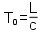time dilation

When our clock moves at the speed v, travelling a distance of vT in a time T, light pulse direction is changed and light pulse travels a longer distance than the pulse in a clock at rest. Because the speed of light is constant, light pulse needs more time to reach second mirror.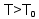Thus, from Pythagorean theorem we can write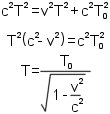Because the light pulse needs more time to reach second mirror, the clock counts slower.

In addition to the time dilation related to the motion (relative velocity) there is also gravitational time dilation. Gravitational time dilation is a consequence of special relativity in accelerated frames of reference. In general relativity, it is considered to be a difference in the passage of proper time at different positions as described by a metric tensor of spacetime.

Time dilation - Wikipedia

In physics, the twin paradox is a thought experiment in special relativity involving identical twins, one of the twins makes a space journey at a speed close to the speed of light and when returns home he find that the twin who stayed on Earth is older. This result appears puzzling because each twin sees the other twin as moving, and so, according to an incorrect naive application of time dilation and the principle of relativity, each should paradoxically find the other to have aged more slowly. However, this scenario can be resolved within the standard framework of special relativity: the travelling twin's trajectory involves two different inertial frames, one for the outbound journey and one for the inbound journey, and so there is no symmetry between the spacetime paths of the two twins. Therefore the twin paradox is not a paradox in the sense of a logical contradiction.

Twin paradox - Wikipedia

Has it been confirmed?

Yes, two scientists took four atomic clocks and set them to be at exactly the same time. They flew twice around the world, first eastward, then westward, and compared the clocks against others that remained at ground. When they got back, they found out that clocks disagree with one another, and their differences were consistent with the predictions of special and general relativity.

Clocks on the ISS space station run slightly slower than reference clocks on Earth, while clocks on GPS and Galileo satellites run slightly faster.

Hafele-Keating experiment - Wikipedia

Time dilation experiment - YouTube

Modern tests